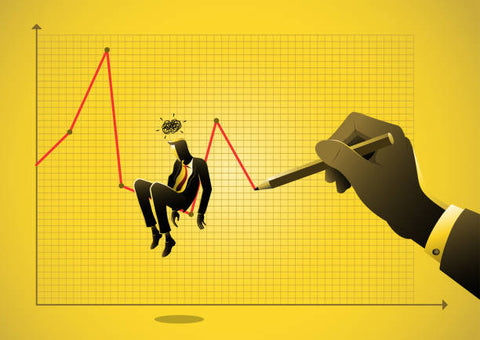## Stock Region News## How To Use Fibonacci Levels When Trading Stocks

Fibonacci levels are a popular tool that traders use to help identify potential support and resistance levels in the market. The Fibonacci sequence is a set of numbers that starts with 0 and 1, and each subsequent number is the sum of the previous two. Fibonacci levels are derived from these numbers and are used by traders to predict areas where the market may find support or resistance. In this article, we'll explain how Fibonacci levels are calculated and show you how to use them when trading stocks.

Source: https://stockregion.com/### What are Fibonacci Levels?

Fibonacci levels are a very popular tool that many traders use to help them make decisions about where to enter and exit trades. The Fibonacci sequence is a set of numbers that starts with 0 and 1, and each subsequent number is the sum of the previous two numbers. So, the sequence goes 0, 1, 1, 2, 3, 5, 8, 13, 21, 34, 55, 89, 144, 233, 377, 610, 987, 1597, 2584, 4181, 6765, 10946, 17711, 28657, 46368, 75025, 121393, 196418, 317811... and so on. Fibonacci levels are derived from this sequence and are used by traders to identify potential support and resistance levels.### How to Use Fibonacci Levels

Fibonacci levels are a popular tool that traders use to help them make decisions about where to enter and exit trades. The Fibonacci sequence is a series of numbers where each number is the sum of the previous two numbers. The most popular Fibonacci levels are 23.6%, 38.2%, 50%, 61.8%, and 100%.

To use Fibonacci levels when trading stocks, you first need to identify the most recent swing high and swing low. A swing high is the highest point that the stock price reaches before it starts to move down again, and a swing low is the lowest point that the stock price reaches before it starts to move up again. Once you have identified the most recent swing high and swing low, you can then calculate the Fibonacci levels.

The 23.6% Fibonacci level is calculated by taking the difference between the swing high and swing low and multiplying it by 0.236. The 38.2% Fibonacci level is calculated by taking the difference between the swing high and swing low and multiplying it by 0.382. The 50% Fibonacci level is simply the midpoint between the swing high and swing low.### Fibonacci Levels and Stock Trading

Fibonacci levels are important technical indicators that can be used to predict stock price movements. Fibonacci levels are based on the Fibonacci sequence, which is a series of numbers where each number is the sum of the previous two numbers. The most important Fibonacci levels for stock trading are the 0.618, 1.0, and 1.618 levels. These levels can be used to predict support and resistance levels, and they can also be used to identify trend reversals.

When using Fibonacci levels for stock trading, it is important to remember that these levels are not exact predictions. Rather, they are guidelines that can be used to help you make better trading decisions. It is also important to use other technical indicators in conjunction with Fibonacci levels, as they can provide additional information that can help you make more informed trading decisions.### What are the Different Types of Fibonacci Levels?

There are several different types of Fibonacci levels that traders use when analyzing stocks. The most common levels are the 0.0%, 23.6%, 38.2%, 50.0%, 61.8%, and 100.0% levels. These levels are derived from the Fibonacci sequence, which is a series of numbers where each number is the sum of the previous two numbers. For example, the Fibonacci sequence starts with 0, 1, 1, 2, 3, 5, 8, 13, 21, 34, 55, 89, 144, 233, etc. As you can see, each number in the sequence is the sum of the previous two numbers.### How to Use Fibonacci Retracement Levels

Fibonacci retracement levels are horizontal lines that indicate where support and resistance are likely to occur. They are based on the Fibonacci sequence, which is a series of numbers where each number is the sum of the two previous numbers. The most popular Fibonacci levels are 23.6%, 38.2%, 50%, 61.8%, and 100%. To use Fibonacci retracement levels, you first need to identify the major trend. Once you have identified the trend, you can then look for pullbacks to these Fibonacci levels. If the stock rallies after retracing to a Fibonacci level, this is a sign that the stock is still in an uptrend. However, if the stock falls below a Fibonacci level, this is a sign that the trend may be reversing.

Source: https://stockregion.com/